# Inline Weir Flow

Weir flow is automatically computed for Inline Weir sections. The program employs the standard weir equation as described below. If the weir structure width (b) is less than the one-half the flow depth (H), it is considered a sharp crested weir. Otherwise, it’s treated as a broad crested weir.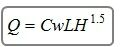Where:

Q = Weir flow
Cw = Broad-crested weir coefficient = 2.66 (1.5)
Cw = Sharp-crested weir coefficient = 3.33 (1.84)
L = Crest width
H = Water surface – crest elevation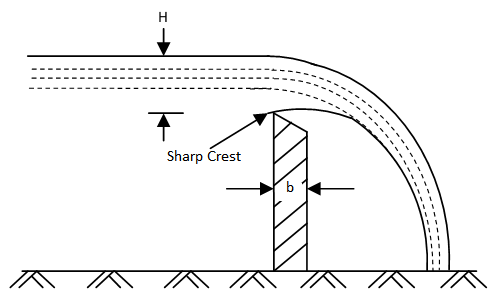Sharp-crested weir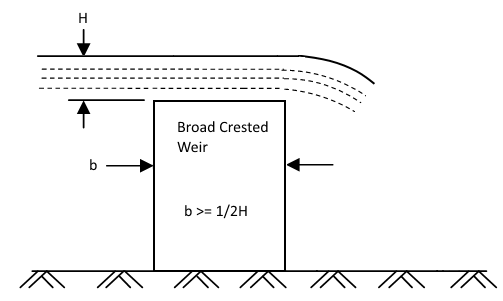Broad-crested weir

### Adjustment for Submerged Weirs

Weirs can be affected by submergence, i.e., when the tailwater or downstream water surface rises above the weir’s crest, thus reducing the discharge. The weir flow Q computed from the above equation is reduced by the following: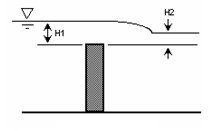The equation for the reduction in flow is: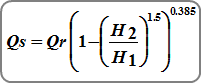Where:
Qs = submerged flow (cfs)
Qr = unsubmerged flow from standard weir equations
H1 = upstream head above crest (ft)
H2 = downstream head above crest (ft)

Updated on October 30, 2018